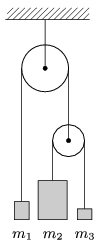Mathematical and Physical Journal
for High Schools
Issued by the MATFUND Foundation
 Already signed up? New to KöMaL?

# KöMaL Problems in Physics, October 2009

Show/hide problems of signs:## Problems with sign 'M'

Deadline expired on November 10, 2009.

M. 299. Measure the coefficient of dynamic friction between a sponge and a sheet of glass in case of different forces with which the sponge and the glass are pushed together. Investigate the dry and the wet sponges as well.

(6 pont)

statistics## Problems with sign 'P'

Deadline expired on November 10, 2009.

P. 4184. What is the temperature measured in Celsius degrees when the Reaumur and the Fahrenheit thermometers show the same value and what is this value?

(3 pont)

solution (in Hungarian), statistics

P. 4185. A bird can fly in the air at a constant speed v. There is a wind of speed v1 blowing from the North. Into what direction should the bird fly in order to move in the East--West direction? What is its speed with respect to the Earth? Data:,.

(3 pont)

solution (in Hungarian), statistics

P. 4186. When someone travels with the underground is it easier to walk facing towards the engine or facing backwards the engine when the train starts to move. Why?

(4 pont)

solution (in Hungarian), statistics

P. 4187. How do the objects shown in the figure move, if m1=2 kg, m2=8 kg, m3=1 kg? The mass of the pulley and the ropes is negligible.(5 pont)

solution (in Hungarian), statistics

P. 4188. A razor blade is floating on the surface of water in a glass. The blade is made sink by moving the glass. How does the level of the water change during this?

(5 pont)

solution (in Hungarian), statistics

P. 4189. 10 cm3 water is poured into a container of volume 400 cm3 at normal atmospheric pressure and at room temperature (20 oC). Then the container is closed hermetically and heated to a temperature of 150 oC. By what amount will the volume of the water change? (Do not consider the change in the volume of the container.)

(4 pont)

solution (in Hungarian), statistics

P. 4190. A cube shaped room of sides 4 m is heated by a stove of power 6 kW. The outside temperature is -10 oC. To what temperature will the room be heated if the coefficient of heat transfer is 2.1?

(4 pont)

solution (in Hungarian), statistics

P. 4191. A sample of Helium gas is cooled down at a pressure of 105 Pa. The gas gives off 5 kJ heat. By how many litres did the volume of the gas decrease?

(4 pont)

solution (in Hungarian), statistics

P. 4192. The metal (hollow) spheres of radii R, 2R and 3R are placed into each other such that their centres are at the same point. The inner sphere is given a charge of Q, the middle one is charged to 2Q, and the outer one is charged to 3Q. a) Find the potentials, measured from the common centre of the circles, at a distances of R, 2R, 3R and 4R, if the potential at the centre is taken to be zero. b) What is the energy of the electric field in the regions between the spheres and outside the spheres? (R=10 cm, Q=2.10-6 C.)

(5 pont)

solution (in Hungarian), statistics

P. 4193. What is the distance between the optical axis of a glass sphere of radius R and that light ray which travels parallel to the optical axis and which leaves the glass at the point where the optical axis intersects the sphere? (The refractive index is 1.6.)

(4 pont)

solution (in Hungarian), statistics